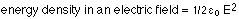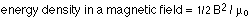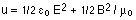# HOW WE CAN SAY THAT EM WAVES CARRY BOTH MOMENTUM AND ENERGY ...?

Electromagnetic waves like any other wave carry energy as they travel through empty space. If light didn't carry energy, it wouldn't be able to heat stuff up or generate photo current in photo cells.

------

As we know that em waves have mutually perpendicular oscillating electric and magnetic fields. There is an energy density associated with both the electric and magnetic field. In general, the energy per unit volume in an electric field is given by:In a magnetic field, the energy per unit volume is:So the total energy density associated with an electromagnetic wave is:It is obvious that energy density is proportional to the square of the amplitudes of electric and magnetic field. Another quantity Intensity measures the rate of transfer of em wave energy per unit area. Intensity = energy density multiplied by velocity of wave. Intensity also depends on the square of amplitude.

---

However according to Quantum Mechanics, quantization of radiation energy has been proposed. An em wave is assumed to be consists of small descrete packets of energy called 'photons'. The energy of photon is proportional to the frequency of wave by Max Plank equation. However increasing the amplitude of em wave increases the energy density(or intensity) which means now there are more number of photons.

----

Difference between energy density of wave and energy of photon becomes apparent in things like the photoelectric effect, in which incoming photons cause electrons to be knocked loose. Increasing the frequency of the light increases the energy of each photon which in turn increases the energy of ejected electron, and increasing its amplitude only increases the number of photons which cause more number of photo electronic emissions.

----------------------------------

We have seen that electromagnetic waves carry energy. It turns out that they also carry momentum and exert radiation pressure. Momentum can be thought of as an object's ability to push another object due to its motion. It is seen in photoelectric effect and Compton effect that light has quantized energy and carry momentum even though it is massless. The ejection of electron in photoelectronic effect is a result of collision between two particles; electron and photon.

We can show that the momentum of a photon is equal to the energy of photon divided by its velocity.

p = E/c

-----

There is well-known relativistic Energy-momentum formula

E 2 = p 2c2 + (m 0c2)2 --- (1)

---

We know that Relativistic mass = Rest mass / (1 - v2 /c2 )1/2

mrel = m0 / (1 - v2 /c2 )1/2

For photon that travels with a velocity 'c' . It's rest mass m0 will be zero.

Therefore for a photon with momentum p and zero rest mass. equation (1) reduces to

E = pc

p = E / c

Using E = hc /λ (Planck's equation)

E = hc / λ = pc

which gives

p = h/λ

We expect particles with mass to have momentum, but now we see that massless particles including photons also carry momentum. But a photon momentum is very small, since p=h/λ and h is very small. It is for this reason that we do not ordinarily observe photon momentum. Our mirrors do not recoil when light reflects from them. However radiation pressure can be measured with sensitive instruments such as Nichols radiometer.

• -18
What are you looking for?